Home » 4 Unique Methods to Optimize your Python Code for Data Science

# 4 Unique Methods to Optimize your Python Code for Data Science

## Overview

• Writing optimized Python code is a crucial piece in your data science skillset
• Here are four methods to optimize your Python code (with plenty of examples!)

## Introduction

I’m a programmer at heart. I’ve been doing programming since well before my university days and I continue to be amazed at the sheer number of avenues that open up using simple Python code.

But I wasn’t always efficient at it. I believe this is a trait most programmers share – especially those who are just starting out. The thrill of writing code always takes precedence over how efficient and neat it is. While this works during our college days, things are wildly different in a professional environment, especially a data science project.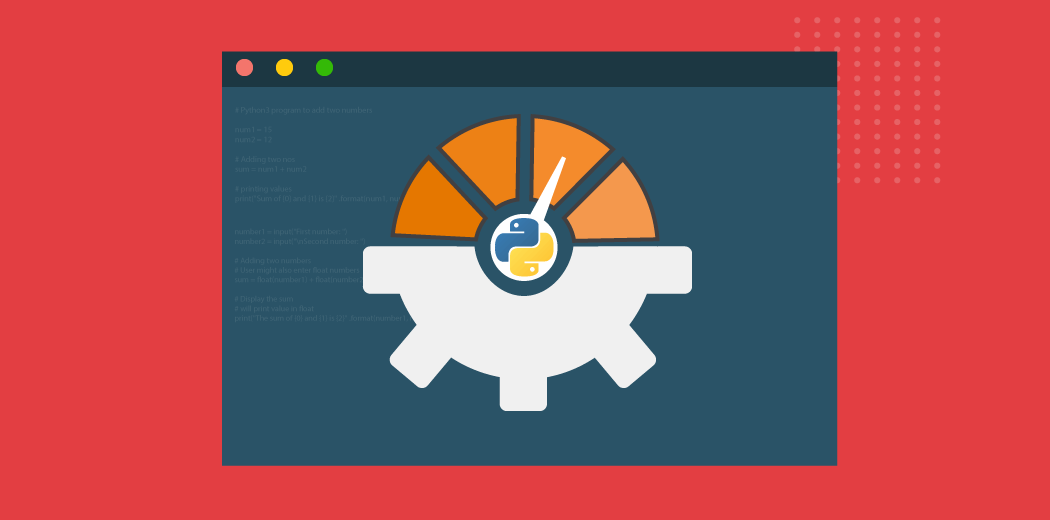Writing optimized Python code is very, very important as a data scientist. There are no two ways about it – a messy, inefficient notebook will cost you time and your project a lot of money. As experienced data scientists and professionals know, this is unacceptable when we’re working with a client.

So in this article, I draw on my years of experience in programming to list down and showcase four methods you can use to optimize Python code for your data science project.

If you’re new to the world of Python (and Data Science), I recommend going through the below resources:

## What is Optimization?

Let’s first define what optimization is. And we’ll do this using an intuitive example.

Here’s our problem statement:

Suppose we are given an array where each index represents a city and the value of that index represents the distance between that city and the next city. Let’s say we have two indices and we need to calculate the total distance between those two indices. In simple terms, we need to find the total sum between any two given indices.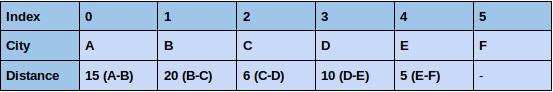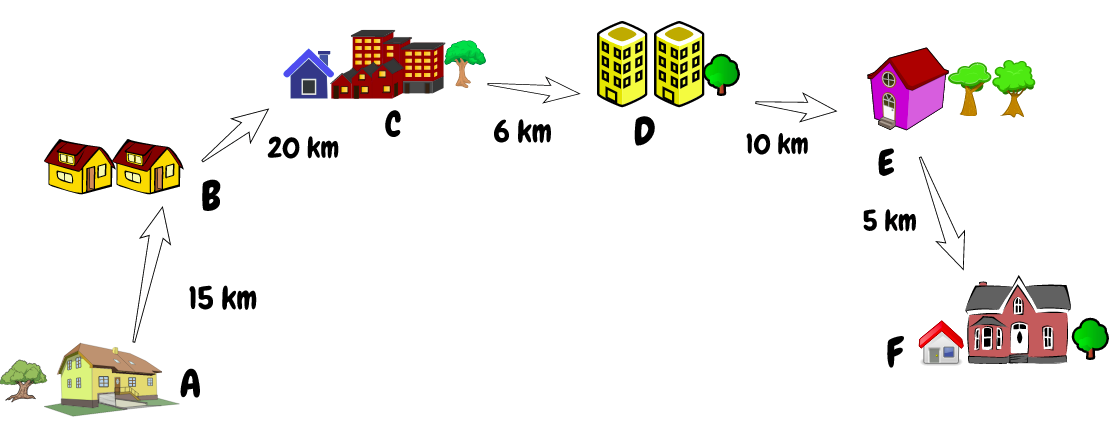The first thought that comes to mind is that a simple FOR loop will work well here. But what if there are 100,000+ cities and we are receiving 50,000+ queries per second? Do you still think a FOR loop will give us a good enough solution for our problem?

Not really. And this is where optimizing our code works wonders.

Code optimization, in simple terms, means reducing the number of operations to execute any task while producing the correct results.

Let’s calculate the number of operations a FOR loop will take to perform this task: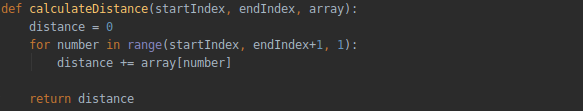We have to figure out the distance between the city with index 1 and index 3 in the above array.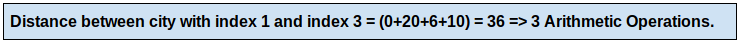What if the array size is 100,000 and the number of queries is 50,000?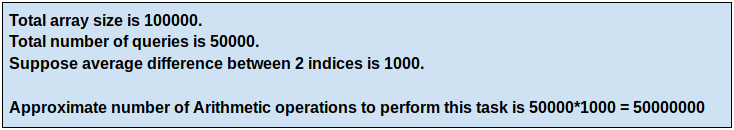This is quite a massive number. Our FOR loop will take a lot of time if the size of the array and the number of queries are further increased. Can you think of an optimized method where we can produce the correct results while using a lesser number of solutions?

Here, I will talk about a potentially better solution to solve this problem by using the prefix array to calculate the distances. Let’s see how it works: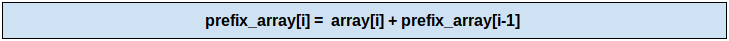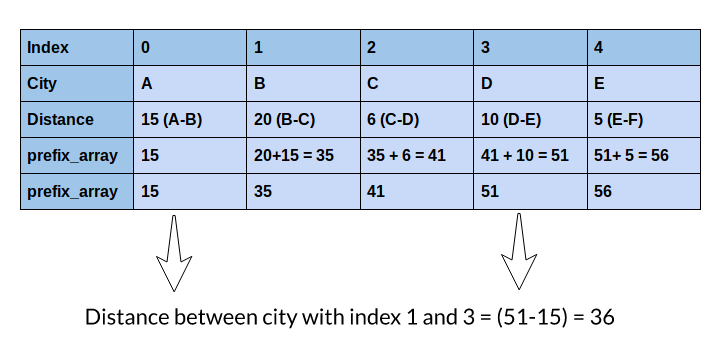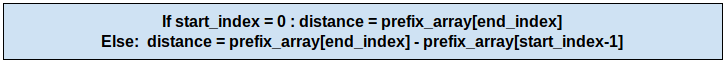Can you understand what we did here? We got the same distance with just one operation! And the best thing about this method is that it will take just one operation to calculate the distance between any two indices, regardless of if the difference between the indices is 1 or 100,000. Isn’t that amazing?

I have created a sample dataset with an array size of 100,000 and 50,000 queries. Let’s compare the time taken by both the methods in the live coding window below.

Note: The dataset has a total of 50,000 queries and you can change the parameter execute_queries to execute any number of queries up to 50,000 and see the time taken by each method to perform the task.

This is the importance and power of optimizing your Python code. We not only save time by getting things done a lot faster, but we also save a lot of computational power too!
You might be wondering how all of this applies to data science projects. Well, you might have noticed that a lot of the time we have to execute the same query on a large number of data points. This is especially true during the data pre-processing stage.
It’s essential that we use some optimized techniques instead of basic programming to get things done as quickly and efficiently as possible. So, here I will share some of the best techniques that I use to improve and optimize my Python code.

## 1. Pandas.apply() – A Feature Engineering Gem

Pandas is already a highly optimized library but most of us still do not make the best use of it. Think about the common places in a data science project where you use it.

One function I can think of is Feature Engineering where we create new features using existing features. One of the most effective ways to do this is using Pandas.apply().

Here, we can pass a user-defined function and apply it to every single data point of the Pandas series. It is one of the best add-ons to the Pandas library as this function helps to segregate data according to the conditions required. We can then efficiently use it for data manipulation tasks.

Let’s use the Twitter sentiment analysis data to calculate the word count for each tweet. We will be using different methods, like the dataframe iterrows method, NumPy array, and the apply method. We’ll then compare it in the live coding window below. You can download the data set from here.

You might have noticed that the apply function is much faster than the iterrows function. Its performance is comparable to the NumPy array but the apply function provides much more flexibility. You can read more about its documentation here.

## 2. Pandas.DataFrame.loc – A Brilliant Hack for Data Manipulation in Python

This is one of my favorite hacks of the Pandas library. I feel this is a must-know method for data scientists who deal with data manipulation tasks (so almost everyone then!).

Most of the time we are required to update only some values of a particular column in a dataset based upon some condition. Pandas.DataFrame.loc gives us the most optimized solution for these kinds of problems.

Let’s solve a problem using this loc function. You can download the dataset we’ll be using here.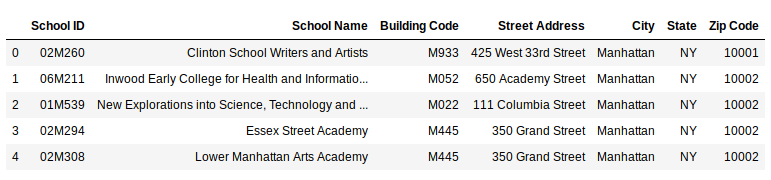Check the value counts of the ‘City’ variable: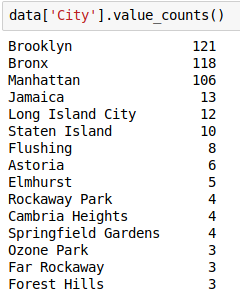Now, let’s say we want only the top 5 cities and want to replace the rest of the cities as ‘Others’. So let’s do that: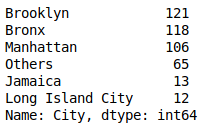See how easy it was to update the values? This is the most optimized way to solve a data manipulation task of this kind.

## 3. Vectorize your Functions in Python

Another way to get rid of slow loops is by vectorizing the function. This means that a newly created function will be applied on a list of inputs and will return an array of results. Vectorizing in Python can speed up your computation by at least two iterations.

Let’s verify this in the live coding window below on the same Twitter Sentiment Analysis Dataset.

Incredible, right? For the above example, vectorization is 80 times faster! This not only helps to speed up our code but also makes it cleaner.

## 4. Multiprocessing in Python

Multiprocessing is the ability of a system to support more than one processor at the same time.
Here, we break our process into multiple tasks and all of them run independently. Even the apply function looks slow when we are working with huge datasets.
So, let’s see how can we make use of the multiprocessing library in Python and speed things up.
We will create one million points at random and calculate the number of divisors for each point. We will compare its performance using both the apply function and the multiprocessing method:
Here, multiprocessing generates the output 13 times faster than the apply method. The performance might vary with different hardware systems but it will definitely improve the performance.

## End Notes

This is by no means an exhaustive list. There are many other methods and techniques to optimize Python code. But I’ve found and used these four a LOT during my data science career and I believe you’ll find them useful too.
Are there any other methods you use to optimize your code? Do share those with us and the community in the comments section below!
And as I mentioned earlier, you should check out our popular courses if you’re new to Python and data science:
You can also read this article on our Mobile APP•ajay says: